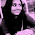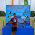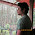VHDL coding tips and tricks: VLSI Interview Questions - Part 2

## Wednesday, April 14, 2010

### VLSI Interview Questions - Part 2

This is part -2 of the interview questions series.Hope it is useful.

1)For the circuit shown below, what should the function F be, so that it produces an output of the same frequency (function F1), and an output of double the frequency (function F2).

a. F1= NOR gate and F2= OR gate
b. F1=NAND gate and F2= AND gate
c. F1=AND gate and F2=XOR gate
d. None of the above
Ans : (d) . (Hint : Assume a small delta delay in the NOT gate).

2)The maximum number of minterms realizable with two inputs (A,B) is:
Ans : For n bits the max number minterms is, (2^n).
For n=2, no. of minterms = (2^2) =  4.
http://www.iberchip.net/VII/cdnav/pdf/75.pdf

3)The maximum number of boolean expressions with two inputs (A,B) is:
Ans : For n bits the max number boolean expressions are, 2^(2^n).
For n=2, no. of boolean expressions = 2^(2^2) =  2^4 = 16.
http://www.iberchip.net/VII/cdnav/pdf/75.pdf

4) A ring counter that counts from 63 to 0 will have ______ D flip-flops,
but a binary counter that counts from 63 to 0 will have _____ D flip-flops
Ans : For ring counter 64. for binary counter 6.

5) Why cache memory is used in computers?
Cache memory is used to increase the speed of memory access by processor.Unlike the main(physical) memory cache memory is small and has very short access time.The most recent data accessed by processor is stored in cache memory.This will help the processor to save time bacause time is not wasted in accessing the same data from the main memory again and again.
A good example is that ,if processor is executing a loop 1000 times involving many variables(so that the CPU registers available are all used up) then the value of these variables can be stored in cache memory.This will make the loop execution faster.
In designing cache, cache miss probability and hit probability determines the efficiency of the cache and the extend to which the average memory access time can be reduced.

6) How will you design a sequence detector?
See this link:
http://web.cs.mun.ca/~paul/cs3724/material/web/notes/node23.html

7) What is setup time and holdtime?
Setup time is the minimum amount of time before the clock’s active edge by which the data must be stable for it to be detected correctly. Any violation in this will cause incorrect data to be captured.
(Analogy for setup time: Suppose you have to catch a train and the train leaves at 8:00.Say you live 20 minutes away from the station, when should you leave your house?
Ans : at 7:40 -> set up time is 20 mins in this case)

Hold time is the minimum amount of time after the clock’s active edge during which the data must be stable. Any violation in this required time causes incorrect data to be latched.
(Suppose your friend needs help in boarding the train and train only allows 5 mins for boarding.How long should you stay after you have arrived?
Ans : Atleast 5 mins -> Hold time is 5 mins )
A very good tutorial with examples about setup time and hold time can be found at this link:
http://nigamanth.net/vlsi/2007/09/13/setup-and-hold-times/

8)What is the difference between Moore and Mealy state machines?
Ans : Moore and Mealy state machines are two ways of designing a state machine. Moore state machines are controlled in such a way that the outputs are a function of the previous state and the inputs. However, Mealy state machines are controlled in a way such that the Outputs may change with a change of state OR with a change of inputs.A Moore state machine may require more states(but less complexity) than a Mealy state machine to accomplish the same task.

#### 8 comments:

1.Hi

I read this post 2 times. It is very useful.

Pls try to keep posting.

Let me show other source that may be good for community.

Source: IT interview questions

Best regards
Jonathan.

2.hi

For Question -2
The maximum number of minterms realizable with two inputs (A,B) is:2^n
which comes out to be 4.
There can be only four min terms for the input combinations:(0,0),(0,1),(1,0) and (1,1)
Please correct me if i am wrong.

3.Thanks mishti for noticing the error. I have updated it. See this link for more explanation.
http://www.iberchip.net/VII/cdnav/pdf/75.pdf

4.c will be the correct answer for question no. 1

1.can you explain

5.for the first question, the answer all depends on the delay of the inverter which is not given.. let us assume the delay of the inverter is equal to the some value and XOR the both the inputs, then by drawing the input and inverted input with some delay, and XORing them , you can get the output which is twice the frequency of the input but with non 50 percent duty cycle , similar is for the case of AND gate.
so the answer is C

6.Moore machine is a finite-state machine whose output values are determined solely by its current state
Mealy machine is a finite-state machine whose output values are determined both by its current state and the current inputs.

7.can any one explain me how to capture the pulse in 50Mhz clok which is comming for 100mhz clock